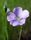## do loops - augmenting numbers

I have attempted various methods, but I have been unable to obtain the desired output.

Using do loops

I need output like following;
1
121
12321
1234321
123454321

1 ACCEPTED SOLUTION

Accepted Solutions

## Re: do loops - augmenting numbers

Is the output one variable? Is it numeric or character? Maximum of 5 or how many rows? (Will severely limit whether the value is numeric or not

This makes a character value with that appearance.

```data example;
length var \$ 25;
do levels = 1 to 5;
call missing(var);
do j=1 to levels;
var=cats(var,j);
end;
do j=(levels-1) to 1 by (-1);
var=cats(var,j);
end;
output;
end;
drop levels j;
run;```
3 REPLIES 3

## Re: doloops

Please show us what you tried, so we can suggest ways to improve/correct your code.LinusH
Tourmaline | Level 20

## Re: do loops - augmenting numbers

Is this some kind of training assignment?
The task doesn't reflect what you normally do using SAS...
Data never sleeps

## Re: do loops - augmenting numbers

Is the output one variable? Is it numeric or character? Maximum of 5 or how many rows? (Will severely limit whether the value is numeric or not

This makes a character value with that appearance.

```data example;
length var \$ 25;
do levels = 1 to 5;
call missing(var);
do j=1 to levels;
var=cats(var,j);
end;
do j=(levels-1) to 1 by (-1);
var=cats(var,j);
end;
output;
end;
drop levels j;
run;```
Discussion stats
• 3 replies
• 180 views
• 1 like
• 4 in conversation